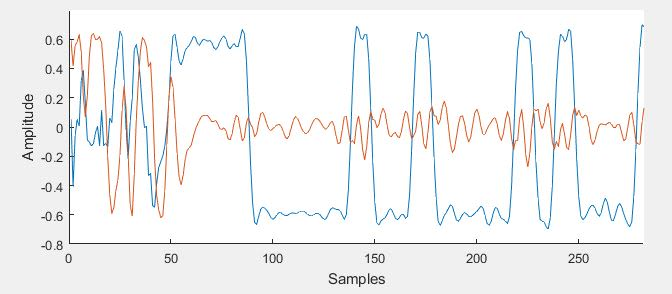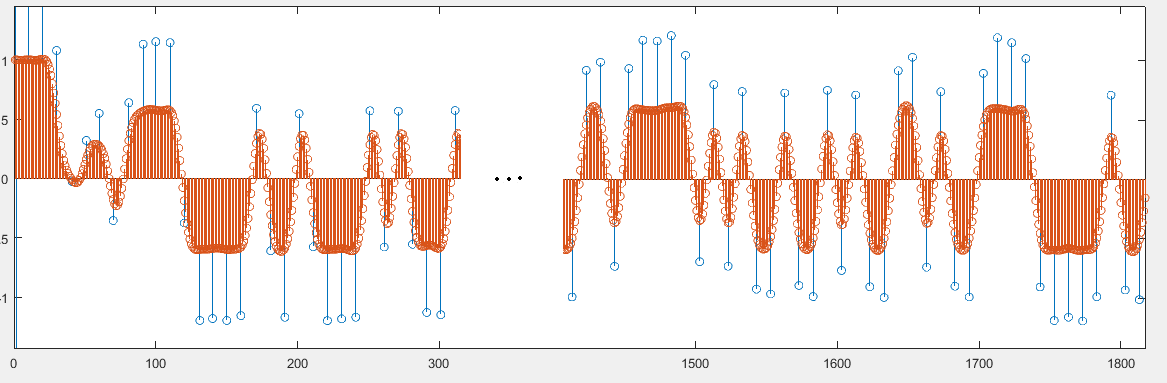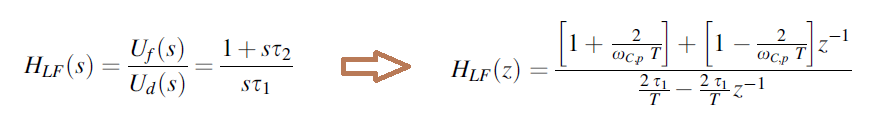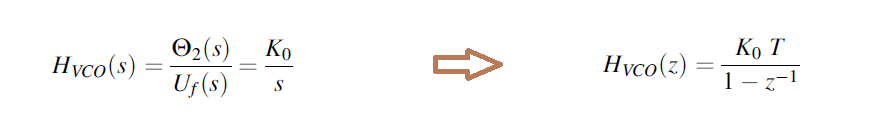About half a year a go I found an excellent book on Costas: https://www.springer.com/gp/book/9783319720074. There are three models of Costas loops for BPSK presented in the book, in-detail explanation of the static and dynamic analysis and mathematical models: conventional circuit on real input, pre-envelope circuit on complex input and finally a phasor rotation circuit on complex input as well. There are explicit mathematical expressions for lock time, lock range, pull-in range, and pull-in time for the Costas loop.

I have implemented the presented pre-envelope model, in Matlab, and using the code that was described in a previous post (https://thinair.aelogic.com/iq-out/) I have fed it with Inmarsat-C signal from one of the channels kindly made available through SDR# spyserver (sdr://pjm.uhf-satcom.com:55555/) at 12 kHz SR.Above, the lock time in samples for a 200 Hz frequency difference.Above, the symbols are also almost centered after 50 samples (~4 ms), and definitely centered not long after that.

At this stage the only hiccup from having an SDR# Inmarsat-C plugin are two transfer functions that I would like to implement as FIR filter approximations in C#. I have found a fast C# FIR implementation at: https://filtering.mathdotnet.com/api/MathNet.Filtering.Channel/index.htm

The first one is the transfer function of the loop filter, this is a “proportional-integral” filter whose transfer function is:and the second one is the VCO “simple integrator” filter having the following transfer function:For my particular case, the loop filter is:

```>> tf(F3_num, F3_den, 1/12000)

ans =

8.916 z - 6.916
---------------
0.48 z - 0.48

Sample time: 8.3333e-05 seconds
Discrete-time transfer function.```

and the VCO:

```>> tf(F4_num, F4_den, 1/12000)

ans =

8.333e-05
---------
z - 1

Sample time: 8.3333e-05 seconds
Discrete-time transfer function.```

Currently I do not know enough math to be able to transform these into FIR coefficients, I am currently trying a FIR from frequency response method. Can this be done?

Any help would be greatly appreciated.